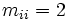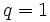# Iwahori-Hecke algebra of a Coxeter group

## Definition

Let$G$ be a Coxeter group with Coxeter presentation:$G := \langle s_1, s_2, \dots, s_n \mid (s_is_j)^{m_{ij}} \rangle$

where$m_{ij} = m_{ji}$ and$m_{ii} = 2$. The Iwahori-Hecke algebra of$G$ over a ring$R$ is defined as the$R[q]$-algebra (for an indeterminate$q$) generated by$T_1, T_2, \dots, T_n$ with the following relations:$(T_i - q)(T_i + 1) = 0$

and the Artin braid relations:$T_iT_j \dots = T_j T_i \dots$,

where the length of both sides is$m_{ij}$. If$m_{ij}$ is even, the left side ends with$T_j$ and the right side ends with$T_i$. Otherwise, the left side ends with$T_i$ and the right side ends with$T_j$.

For specific choices of$q \in R$, we get a$R$-algebra. Thus, the$R[q]$-algebra can be viewed as a one-parameter family of$R$-algebras. When$q = 1$, we get the group ring of$G$ over$R$. To distinguish itself from the algebras obtained by setting particular values of$q$, the Iwahori-Hecke algebra is also sometimes termed the generic Hecke algebra. Choosing a particular value of$q$ is specialization.

## For the Weyl group of a Chevalley group

If$W$ is the Weyl group of a Chevalley group, then$W$ has a natural choice of Coxeter presentation. For this choice of Coxeter presentation, we can define the Iwahori-Hecke algebra as above. It turns out that the Hecke algebra of the Chevalley group realized over a field of size$q$, taken over the ring$R$ is isomorphic to the Iwahori-Hecke algebra described above, specialized at$q$.

### The symmetric group and general linear groups

The Weyl group of the general linear group of order$n$ over any field is the symmetric group of degree$n$. The Iwahori-Hecke algebra of the symmetric group has the property that when specialized to a particular value of$q$, it gives the Hecke algebra of a general linear group of order$n$ over a field of size$q$.